Latest Banking jobs   »   Quantitative Aptitude Quiz For IBPS RRB...

# Quantitative Aptitude Quiz For IBPS RRB PO, Clerk Prelims 2021- 24th May

Direction (1-5): Data given below shows total number of students in three schools i.e, A, B and C.
Total number of students in school A, B and C are 800, 1200 and 900 respectively
p1 , p2, p3 are the number of boys in school A, B and C respectively
q1 , q2, q3 are the number of girls in school A, B and C respectively
Total number of mentors in school A → 20% of boys in school A
Total number of mentors in school B → 30% of girls in school B
Total number of mentors in school C → 10% of boys in school C
p1 : q1 → 3 : 1
p2 : q2 → 5 : 3
p3 : q3 → 2 : 1

Q1. Total number of boys in school ‘B’ is what percent more than total number of girls in school ‘C’?
(a) 25%
(b) 150%
(c) 125%
(d) 250%
(e) 60%

Q2. Total number of boys in school ‘A’ is how much more than total number of girls in school ‘B’
(a) 200
(b) 100
(c) 250
(d) 300
(e) 150

Q3. Number of mentors in school ‘B’ is what percent of number of mentors in school ‘C’.
(a) 225%
(b) 125%
(c) 100%
(d) 200%
(e) 250%

Q4. In school ‘D’, total number of girls is 25% more than girls in school ‘A’ while total number of boys is 40% less than number of boys in school ‘B’. Find total number of students in school ‘D’.
(a) 600
(b) 1380
(c) 700
(d) 1020
(e) 1300

Q5. Find the ratio between total number of girls in school ‘B’ to total number of boys in school ‘C’.
(a) 4 : 5
(b) 4 : 3
(c) 5 : 4
(d) 3 : 4
(e) 1 : 2

Direction (6 – 10): Read the data carefully and answer the questions.
In a school ‘X’ there are eight classes i.e. one to eight. Total student in class two is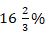more than total students in class one, while number of students in class five is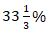less than that of students in class one. Total students in class one, two and five together is 204. Total students in class three is 12 more than total students in class two. Total students in school ‘X’ is 86% more than sum of students in class one, two, three & five together. Total students in class eight is 14 more than total students in class four, while total students in class seven is 4 less than total students in class four. Total students in class six is 22 less than total students in class eight.

Q6. Find average of number of students in class two, three, five and seven?
(a) 64
(b) 56
(c) 84
(d) 72
(e) 96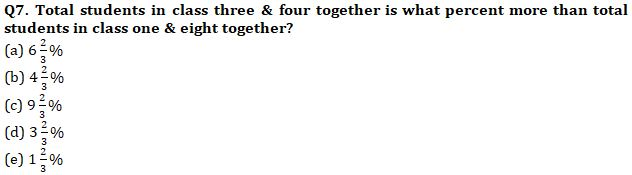Q8. Find the ratio between total students in class five & six together to total students in three & seven together?
(a) 2 : 5
(b) 2 : 7
(c) 2 : 3
(d) 2 : 9
(e) 2 : 11

Q9. If total students in class eight of school ‘Y’ is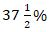more than total students in class three of school ‘X’, then total students in class eight of school ‘Y’ is what percent less than total students in class three & four together of school ‘X’?
(a) 12.5%
(b) 22.5%
(c) 20.5%
(d) 17.5%
(e) 15.5%

Q10. Find total number of students in class one, three, five & seven of school ‘X’?
(a) 256
(b) 276
(c) 284
(d) 302
(e) 316

Directions (11-15): Line chart given below shows percentage of female working in five different companies out of total employees and table given below shows difference between male employees and female employees working in respective company. Study the data carefully and answer the following questions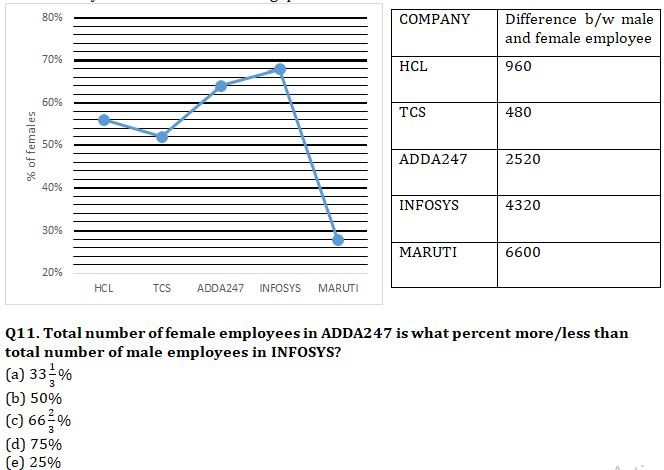Q12. Find the average number of female employees working in HCL and TCS?
(a) 5060
(b) 4840
(c) 5160
(d) 4640
(e) 5360

Q13. Find the difference between female employees working in MARUTI to female employees working in INFOSYS?
(a) 3840
(b) 3900
(c) 3960
(d) 4020
(e) 4080

Q14. In which of the given companies, number of male employees is greater than number of female employees?
(a) MARUTI
(c) HCL
(d) INFOSYS
(e) TCS

Q15. Find the ratio between male employees in ADDA247 to male employees in MARUTI?
(a) 1 : 3
(b) 2 : 9
(c) 1 : 5
(d) 3 : 10
(e) 2 : 5

Practice More Questions of Quantitative Aptitude for Competitive Exams:

###### Study Plan for IBPS RRB PO/Clerk Prelims 2021

Solutions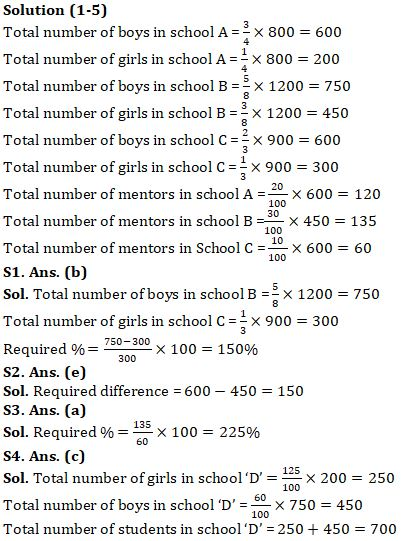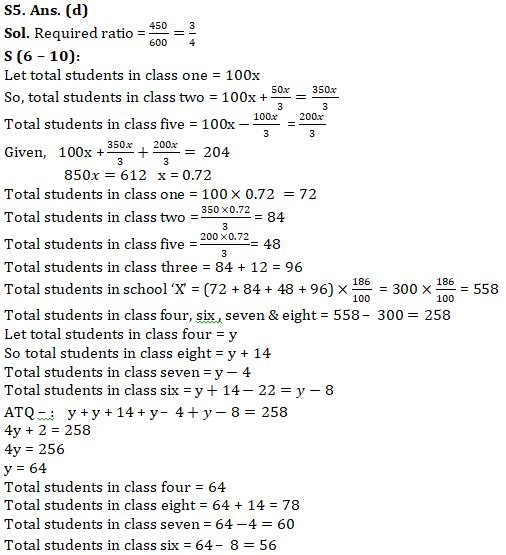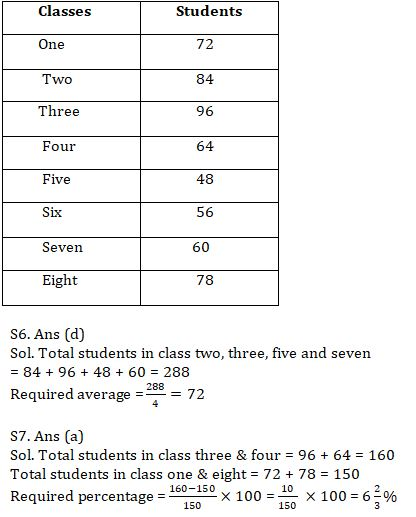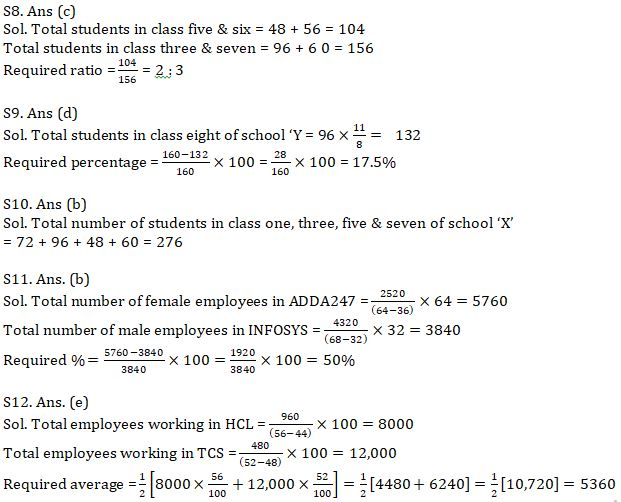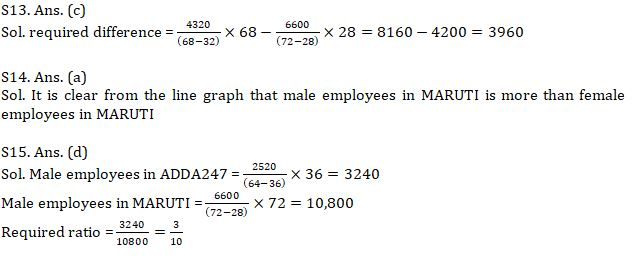#### Congratulations!Incorrect details? Fill the form again here

•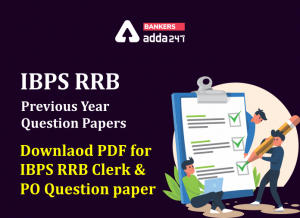IBPS RRB Previous Year Question Paper PD...
•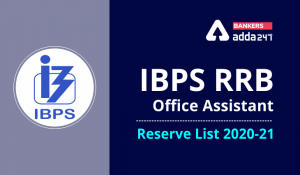IBPS RRB Clerk 2nd Reserve List 2022 Out...
•Quantitative Aptitude Quiz For IBPS RRB ...
•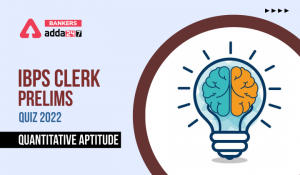Quantitative Aptitude Quiz For IBPS Cler...
•Reasoning Ability Quiz For IBPS RRB PO P...
•Quantitative Aptitude Quiz For IBPS RRB ...